# void main int a32767 printfd a Compiled By

• Slides: 36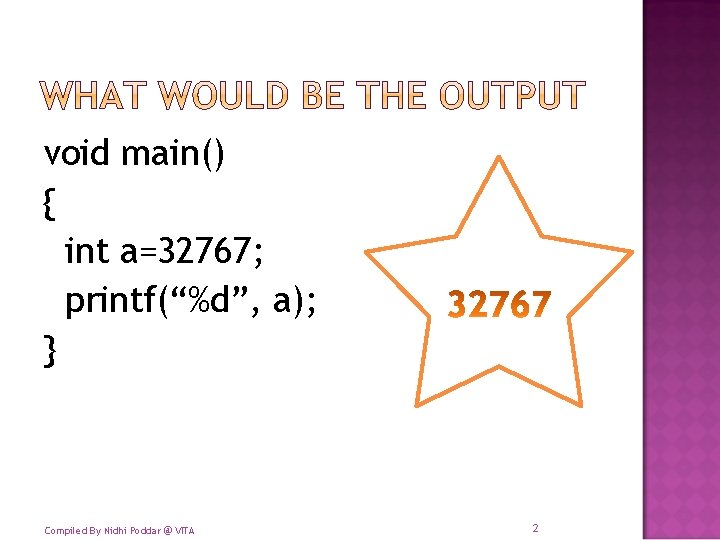void main() { int a=32767; printf(“%d”, a); } Compiled By Nidhi Poddar @ VITA 2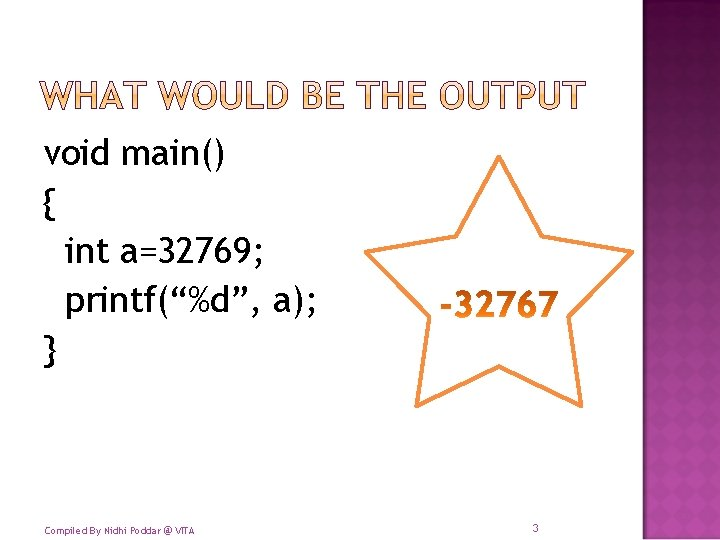void main() { int a=32769; printf(“%d”, a); } Compiled By Nidhi Poddar @ VITA 3void main() { int a=1232. 5; printf(“%d”, a); } Compiled By Nidhi Poddar @ VITA 4void main() { int a=-32779. 205; printf(“%d”, a); } Compiled By Nidhi Poddar @ VITA 5void main() { float a=-3279. 205; printf(“%d”, a); } Compiled By Nidhi Poddar @ VITA 6void main() { int a=-3279. 205; printf(“%f”, a); } Compiled By Nidhi Poddar @ VITA 7void main() { float a=-32779. 205; printf(“%f”, a); } Compiled By Nidhi Poddar @ VITA 8void main() { float a=-32779. 205; printf(“%. 1 f”, a); } Compiled By Nidhi Poddar @ VITA 9void main() { float a=69; printf(“%f”, a); } Compiled By Nidhi Poddar @ VITA 10void main() { int a, b, c; a=5; b=2; c=a/b; printf(“%d”, c); } Compiled By Nidhi Poddar @ VITA 11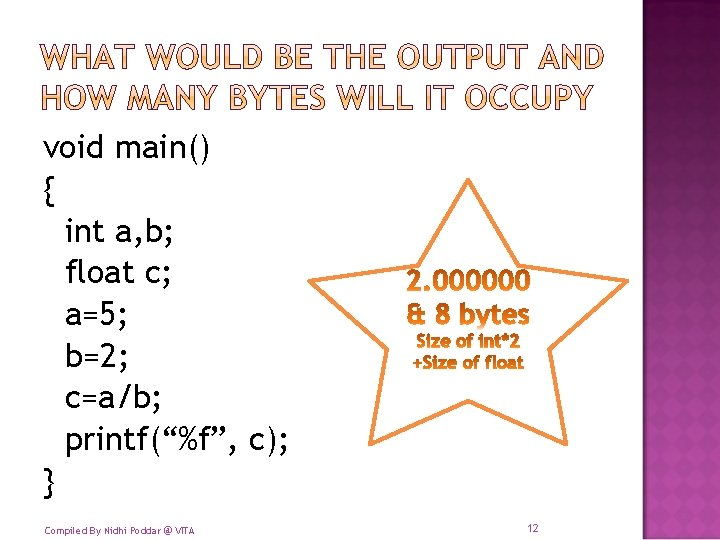void main() { int a, b; float c; a=5; b=2; c=a/b; printf(“%f”, c); } Compiled By Nidhi Poddar @ VITA 12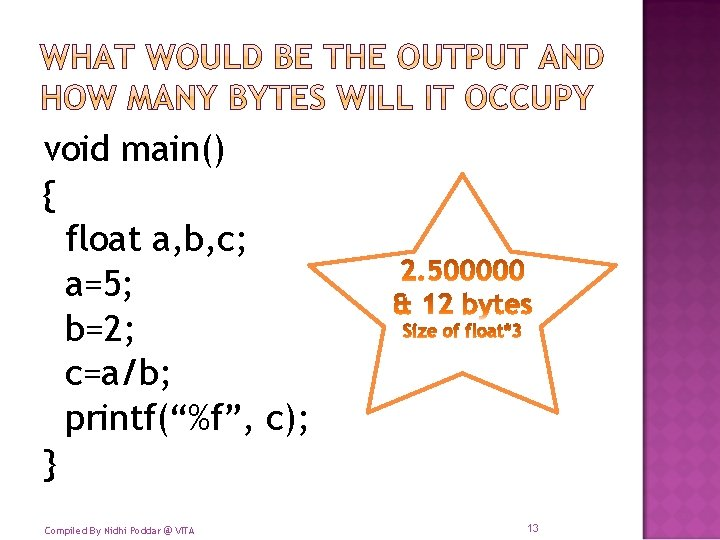void main() { float a, b, c; a=5; b=2; c=a/b; printf(“%f”, c); } Compiled By Nidhi Poddar @ VITA 13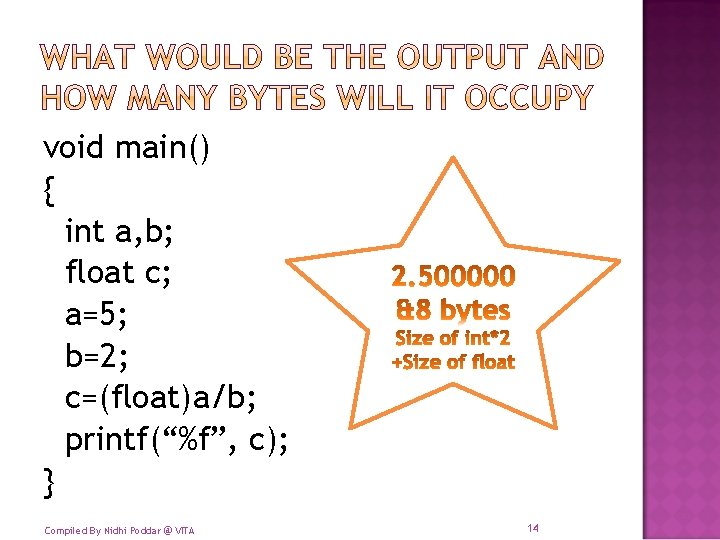void main() { int a, b; float c; a=5; b=2; c=(float)a/b; printf(“%f”, c); } Compiled By Nidhi Poddar @ VITA 14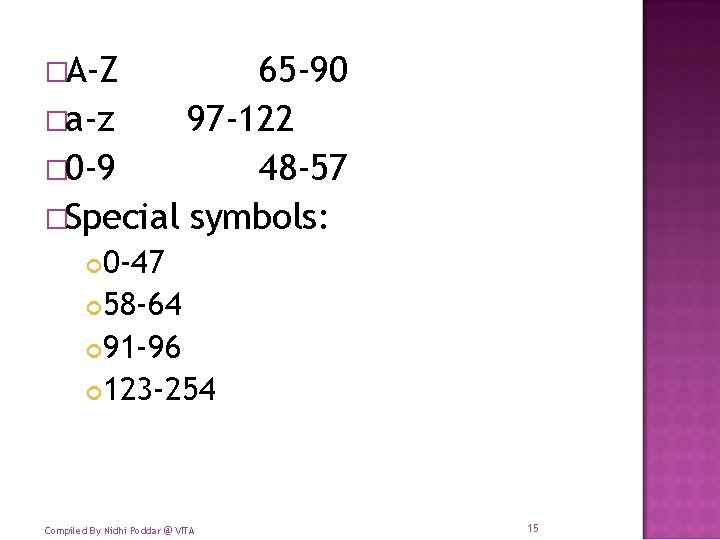�A-Z 65 -90 �a-z 97 -122 � 0 -9 48 -57 �Special symbols: 0 -47 58 -64 91 -96 123 -254 Compiled By Nidhi Poddar @ VITA 15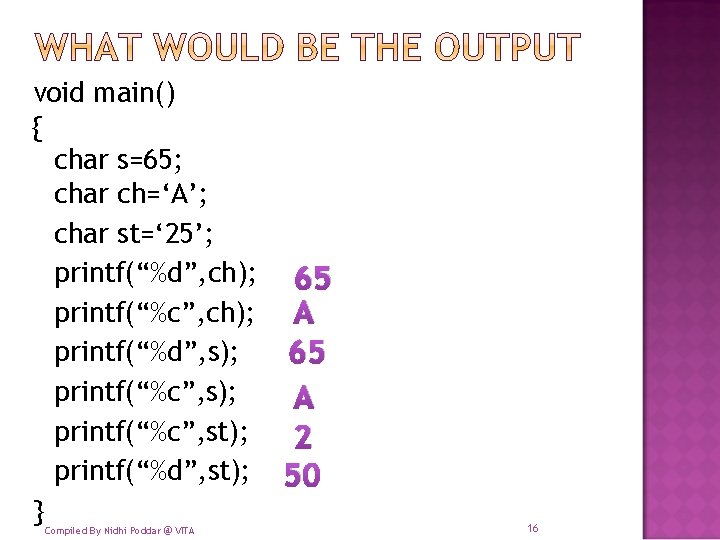void main() { char s=65; char ch=‘A’; char st=‘ 25’; printf(“%d”, ch); 65 printf(“%c”, ch); A printf(“%d”, s); 65 printf(“%c”, s); A printf(“%c”, st); 2 printf(“%d”, st); 50 } Compiled By Nidhi Poddar @ VITA 16� Try some of these: printf(“%c”, -100); £ printf(“%c”, -128); Ç printf(“%c”, -130); ~ printf(“%c”, 100); d printf(“%d”, -10); -10 printf(“%x”, 1>>4); ffff printf(“%x”, 16); 10 Compiled By Nidhi Poddar @ VITA 17� Try some of these: printf(“%d”, -100); printf(“%. 2 f”, 128); printf(“%f”, -130); printf(“%c”, 91); printf(“%d”, 34342); printf(“%x”, 1004); printf(“%x”, 16); Compiled By Nidhi Poddar @ VITA 18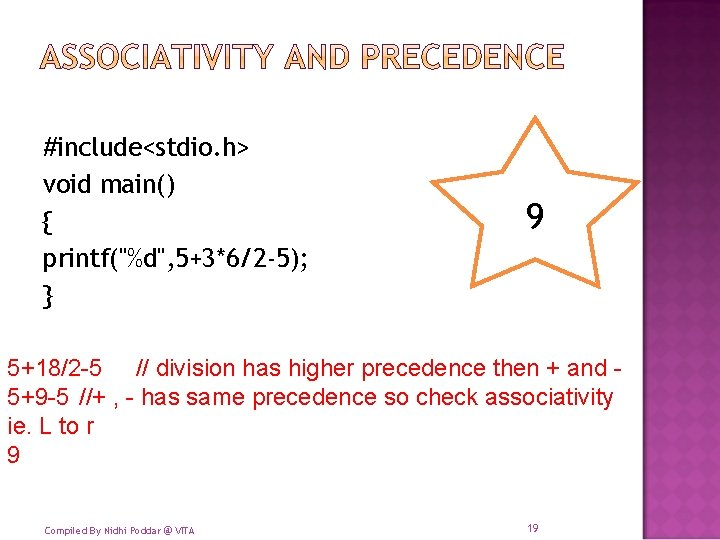#include<stdio. h> void main() { printf("%d", 5+3*6/2 -5); } 9 5+18/2 -5 // division has higher precedence then + and 5+9 -5 //+ , - has same precedence so check associativity ie. L to r 9 Compiled By Nidhi Poddar @ VITA 19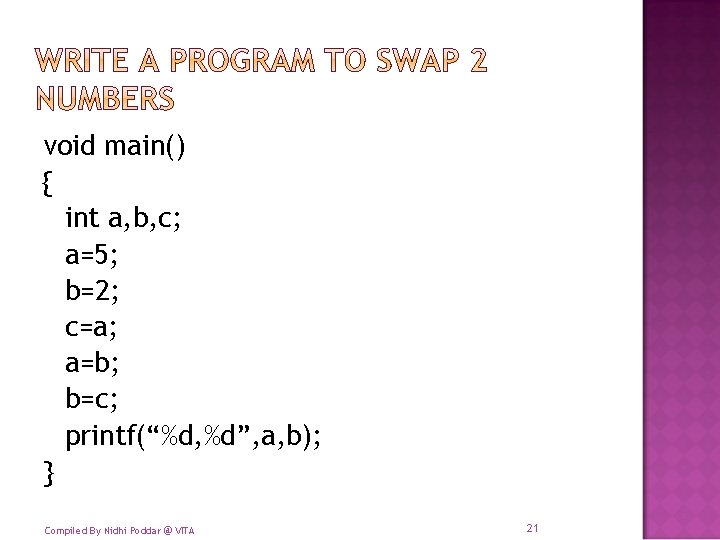void main() { int a, b, c; a=5; b=2; c=a; a=b; b=c; printf(“%d, %d”, a, b); } Compiled By Nidhi Poddar @ VITA 21� Suppose we print c over here what would be its value? 5, as it copy paste the value and does not cut paste the values Compiled By Nidhi Poddar @ VITA 22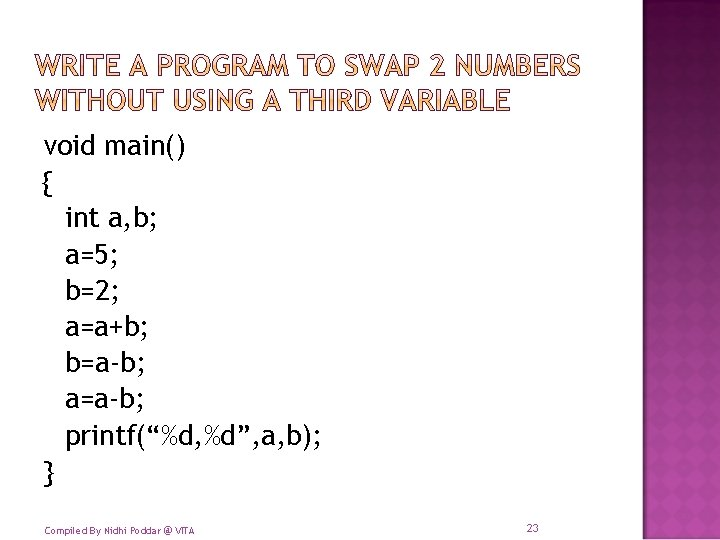void main() { int a, b; a=5; b=2; a=a+b; b=a-b; a=a-b; printf(“%d, %d”, a, b); } Compiled By Nidhi Poddar @ VITA 23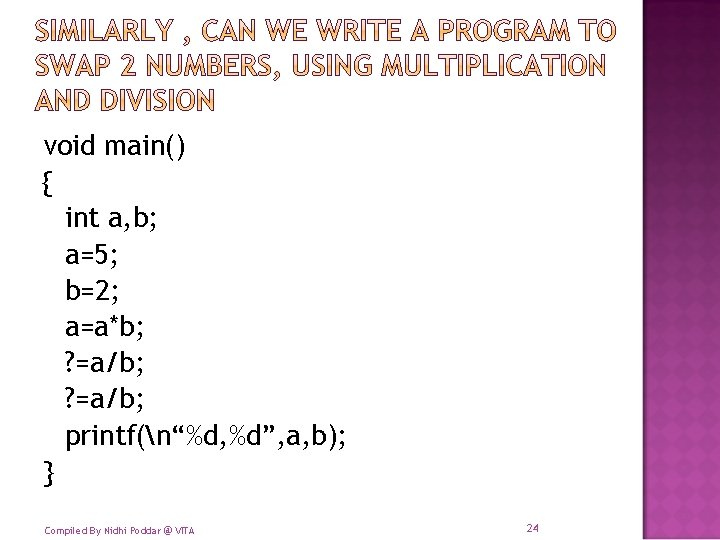void main() { int a, b; a=5; b=2; a=a*b; ? =a/b; printf(n“%d, %d”, a, b); } Compiled By Nidhi Poddar @ VITA 24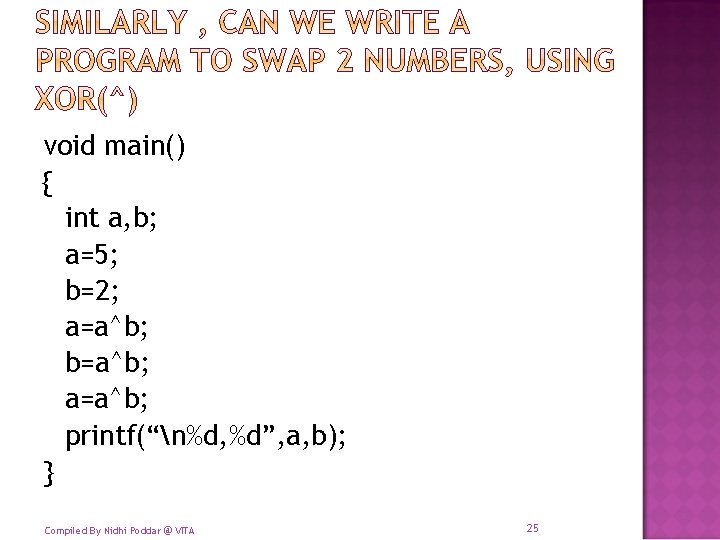void main() { int a, b; a=5; b=2; a=a^b; b=a^b; a=a^b; printf(“n%d, %d”, a, b); } Compiled By Nidhi Poddar @ VITA 25� a=5 � b=6 � a^b=3 � a=3 � b=6 � a^b=5 � a=3 � b=5 � a^b=6 Compiled By Nidhi Poddar @ VITA 101 110 011 110 101 011 101 110 26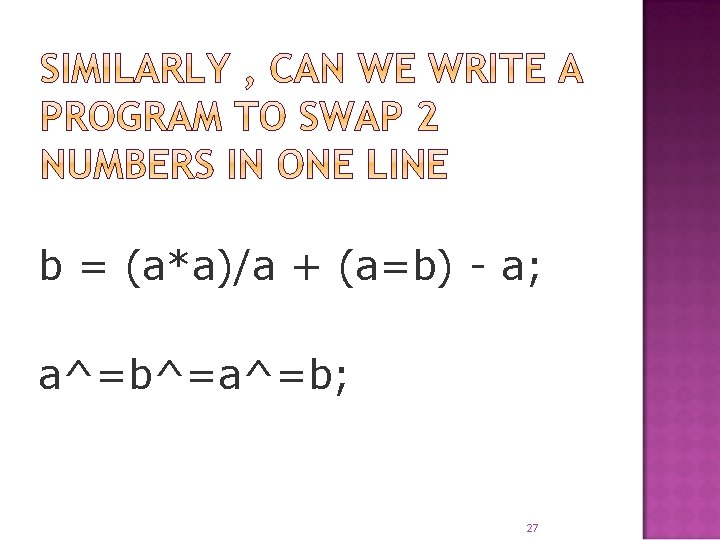b = (a*a)/a + (a=b) - a; a^=b^=a^=b; 272829void main() { char s=5; s++; printf(“%d”, s); printf(“%d”, s++); printf(“%d”, s); printf(“%d”, ++s); printf(“%d”, s); 6 6 7 8 8 } Compiled By Nidhi Poddar @ VITA 30void main() { int s=5; s++; printf(“%d”, s); printf(“%d”, s--); printf(“%d”, s); printf(“%d”, --s); printf(“%d”, s); 6 6 5 4 4 } Compiled By Nidhi Poddar @ VITA 31void main() { char s=5; printf(“%d”, s+++s); } 5+6= 11 What would the compiler read it as? a++ +a Or a+ ++a Note: Post increment has higher precedence than pre increment Compiled By Nidhi Poddar @ VITA 32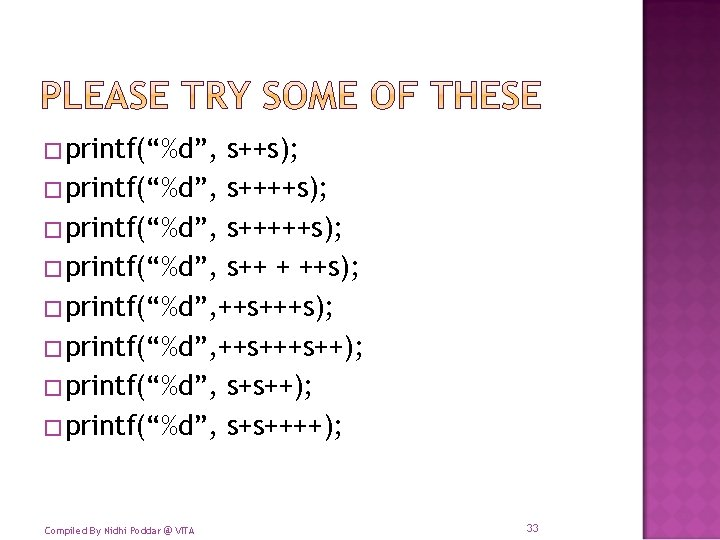�printf(“%d”, s++s); �printf(“%d”, s+++++s); �printf(“%d”, s++ + ++s); �printf(“%d”, ++s+++s++); �printf(“%d”, s+s++++); Compiled By Nidhi Poddar @ VITA 33void main() { char s=5; printf(“%d%d”, ++s, s++, ++s); printf(“n%d”, s); } 9866 9 Note: Post increment has higher precedence than pre increment, but here associativety is Right to Left Compiled By Nidhi Poddar @ VITA 34� 1 km=1000 m � 1 m=100 cm � I inch=2. 54 cm � 1 feet=12 inch Compiled By Nidhi Poddar @ VITA 35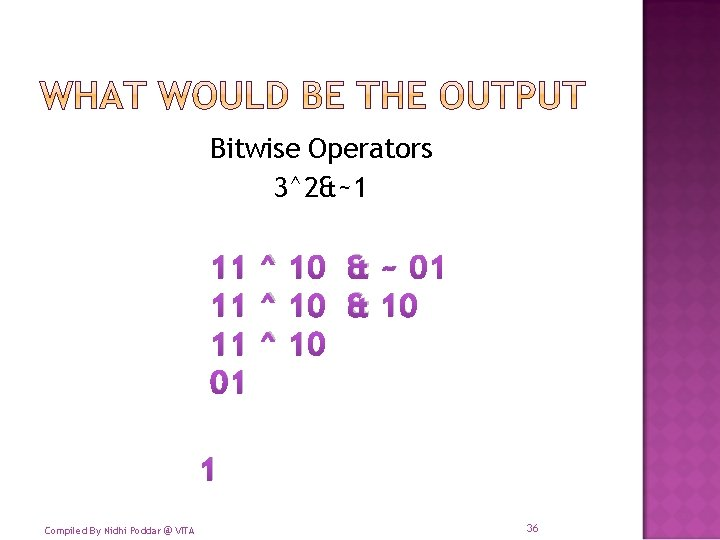Bitwise Operators 3^2&~1 11 ^ 10 & ~ 01 11 ^ 10 & 10 11 ^ 10 01 1 Compiled By Nidhi Poddar @ VITA 36Best of Luck 37